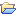# Convolution

This category contains 21 nodes.

##### Keras Convolution 1D Layer

A convolution 1D layer.

##### Keras Convolution 1D LayerDeprecated

A convolution 1D layer.

##### Keras Convolution 2D Layer

A convolution 2D layer.

##### Keras Convolution 2D LayerDeprecated

A convolution 2D layer.

##### Keras Convolution 3D Layer

A convolution 3D layer.

##### Keras Convolution 3D LayerDeprecated

A convolution 3D layer.

##### Keras Cropping 1D Layer

A cropping 1D layer.

##### Keras Cropping 2D Layer

A cropping 2D layer.

##### Keras Cropping 3D Layer

A cropping 3D layer.

##### Keras Separable Convolution 1D Layer

A separable convolution 1D layer.